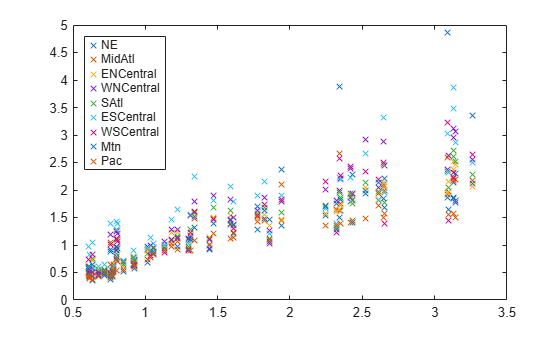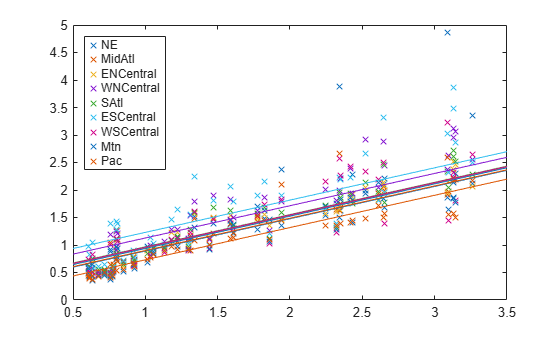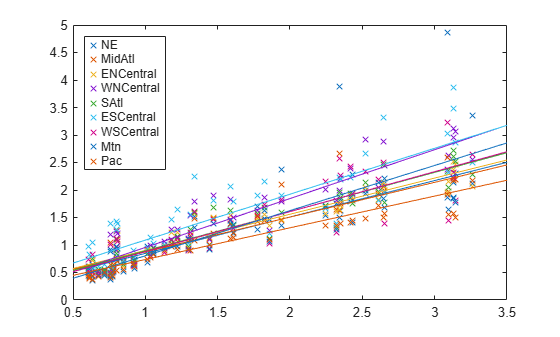Documentation

# mvregress

Multivariate linear regression

## Syntax

``beta = mvregress(X,Y)``
``beta = mvregress(X,Y,Name,Value)``
``````[beta,Sigma] = mvregress(___)``````
``````[beta,Sigma,E,CovB,logL] = mvregress(___)``````

## Description

example

````beta = mvregress(X,Y)` returns the estimated coefficients for a multivariate normal regression of the d-dimensional responses in `Y` on the design matrices in `X`.```

example

````beta = mvregress(X,Y,Name,Value)` returns the estimated coefficients using additional options specified by one or more name-value pair arguments. For example, you can specify the estimation algorithm, initial estimate values, or maximum number of iterations for the regression.```

example

``````[beta,Sigma] = mvregress(___)``` also returns the estimated d-by-d variance-covariance matrix of `Y`, using any of the input arguments from the previous syntaxes.```

example

``````[beta,Sigma,E,CovB,logL] = mvregress(___)``` also returns a matrix of residuals `E`, estimated variance-covariance matrix of the regression coefficients `CovB`, and the value of the log likelihood objective function after the last iteration `logL`.```

## Examples

collapse all

Fit a multivariate regression model to panel data, assuming different intercepts and common slopes.

```load('flu') ```

The dataset array `flu` contains national CDC flu estimates, and nine separate regional estimates based on Google® query data.

Extract the response and predictor data.

```Y = double(flu(:,2:end-1)); [n,d] = size(Y); x = flu.WtdILI; ```

The responses in `Y` are the nine regional flu estimates. Observations exist for every week over a one-year period, so= 52. The dimension of the responses corresponds to the regions, so= 9. The predictors in `x` are the weekly national flu estimates.

Plot the flu data, grouped by region.

```figure; regions = flu.Properties.VarNames(2:end-1); plot(x,Y,'x') legend(regions,'Location','NorthWest') ```Fit the multivariate regression model

``

whereand, with between-region concurrent correlation

``

There are= 10 regression coefficients to estimate: nine intercept terms and a common slope. The input argument `X` should be an-element cell array of-by-design matrices.

```X = cell(n,1); for i = 1:n X{i} = [eye(d) repmat(x(i),d,1)]; end [beta,Sigma] = mvregress(X,Y); ```

`beta` contains estimates of the-dimensional coefficient vector

``

`Sigma` contains estimates of the-by-variance-covariance matrix,for the between-region concurrent correlations.

Plot the fitted regression model.

```B = [beta(1:d)';repmat(beta(end),1,d)]; xx = linspace(.5,3.5)'; fits = [ones(size(xx)),xx]*B; figure; h = plot(x,Y,'x',xx,fits,'-'); for i = 1:d set(h(d+i),'color',get(h(i),'color')); end legend(regions,'Location','NorthWest'); ```The plot shows that each regression line has a different intercept but the same slope. Upon visual inspection, some regression lines appear to fit the data better than others.

Fit a multivariate regression model to panel data using least squares, assuming different intercepts and slopes.

```load('flu'); ```

The dataset array `flu` contains national CDC flu estimates, and nine separate regional estimates based on Google® queries.

Extract the response and predictor data.

```Y = double(flu(:,2:end-1)); [n,d] = size(Y); x = flu.WtdILI; ```

The responses in `Y` are the nine regional flu estimates. Observations exist for every week over a one-year period, so= 52. The dimension of the responses corresponds to the regions, so= 9. The predictors in `x` are the weekly national flu estimates.

Fit the multivariate regression model

``

whereand, with between-region concurrent correlation

``

There are= 18 regression coefficients to estimate: nine intercept terms, and nine slope terms. `X` is an-element cell array of-by-design matrices.

```X = cell(n,1); for i = 1:n X{i} = [eye(d) x(i)*eye(d)]; end [beta,Sigma] = mvregress(X,Y,'algorithm','cwls'); ```

`beta` contains estimates of the-dimensional coefficient vector

``

Plot the fitted regression model.

```B = [beta(1:d)';beta(d+1:end)']; xx = linspace(.5,3.5)'; fits = [ones(size(xx)),xx]*B; figure; h = plot(x,Y,'x',xx,fits,'-'); for i = 1:d set(h(d+i),'color',get(h(i),'color')); end regions = flu.Properties.VarNames(2:end-1); legend(regions,'Location','NorthWest'); ```The plot shows that each regression line has a different intercept and slope.

Fit a multivariate regression model using a single $n$-by-$P$ design matrix for all response dimensions.

`load('flu')`

The dataset array `flu` contains national CDC flu estimates, and nine separate regional estimates based on Google® queries.

Extract the response and predictor data.

```Y = double(flu(:,2:end-1)); [n,d] = size(Y); x = flu.WtdILI;```

The responses in `Y` are the nine regional flu estimates. Observations exist for every week over a one-year period, so $n$ = 52. The dimension of the responses corresponds to the regions, so $d$ = 9. The predictors in `x` are the weekly national flu estimates.

Create an $n$ -by- $P$ design matrix `X`. Add a column of ones to include a constant term in the regression.

`X = [ones(size(x)),x];`

Fit the multivariate regression model

`${y}_{ij}={\alpha }_{j}+{\beta }_{j}{x}_{ij}+{ϵ}_{ij},$`

where $i=1,\dots ,n$ and $j=1,\dots ,d$, with between-region concurrent correlation

`$COV\left({ϵ}_{ij},{ϵ}_{ij}\right)={\sigma }_{jj}.$`

There are 18 regression coefficients to estimate: nine intercept terms, and nine slope terms.

`[beta,Sigma,E,CovB,logL] = mvregress(X,Y);`

`beta` contains estimates of the $P$-by-$d$ coefficient matrix. `Sigma` contains estimates of the $d$-by-$d$ variance-covariance matrix for the between-region concurrent correlations. `E` is a matrix of the residuals. `CovB` is the estimated variance-covariance matrix of the regression coefficients. `logL` is the value of the log likelihood objective function after the last iteration.

Plot the fitted regression model.

```B = beta; xx = linspace(.5,3.5)'; fits = [ones(size(xx)),xx]*B; figure h = plot(x,Y,'x', xx,fits,'-'); for i = 1:d set(h(d+i),'color',get(h(i),'color')) end regions = flu.Properties.VarNames(2:end-1); legend(regions,'Location','NorthWest')```The plot shows that each regression line has a different intercept and slope.

## Input Arguments

collapse all

Design matrices for the multivariate regression, specified as a matrix or cell array of matrices. n is the number of observations in the data, K is the number of regression coefficients to estimate, p is the number of predictor variables, and d is the number of dimensions in the response variable matrix `Y`.

• If d = 1, then specify `X` as a single n-by-K design matrix.

• If d > 1 and all d dimensions have the same design matrix, then you can specify `X` as a single n-by-p design matrix (not in a cell array).

• If d > 1 and all n observations have the same design matrix, then you can specify `X` as a cell array containing a single d-by-K design matrix.

• If d > 1 and all n observations do not have the same design matrix, then specify `X` as a cell array of length n containing d-by-K design matrices.

To include a constant term in the regression model, each design matrix should contain a column of ones.

`mvregress` treats `NaN` values in `X` as missing values, and ignores rows in `X` with missing values.

Data Types: `single` | `double` | `cell`

Response variables, specified as an n-by-d matrix. n is the number of observations in the data, and d is the number of dimensions in the response. When d = 1, `mvregress` treats the values in `Y` like n independent response values.

`mvregress` treats `NaN` values in `Y` as missing values, and handles them according to the estimation algorithm specified using the name-value pair argument `algorithm`.

Data Types: `single` | `double`

### Name-Value Pair Arguments

Specify optional comma-separated pairs of `Name,Value` arguments. `Name` is the argument name and `Value` is the corresponding value. `Name` must appear inside quotes. You can specify several name and value pair arguments in any order as `Name1,Value1,...,NameN,ValueN`.

Example: `'algorithm','cwls','covar0',C` specifies covariance-weighted least squares estimation using the covariance matrix `C`.

Estimation algorithm, specified as the comma-separated pair consisting of `'algorithm'` and one of the following.

 `'mvn'` Ordinary multivariate normal maximum likelihood estimation. `'ecm'` Maximum likelihood estimation via the ECM algorithm. `'cwls'` Covariance-weighted least squares estimation.

The default algorithm depends on the presence of missing data.

• For complete data, the default is `'mvn'`.

• If there are any missing responses (indicated by `NaN`), the default is `'ecm'`, provided the sample size is sufficient to estimate all parameters. Otherwise, the default algorithm is `'cwls'`.

### Note

If `algorithm` has the value `'mvn'`, then `mvregress` removes observations with missing response values before estimation.

Example: `'algorithm','ecm'`

Initial estimates for the regression coefficients, specified as the comma-separated pair consisting of `'beta0'` and a vector with K elements. The default value is a vector of 0s.

The `beta0` argument is not used if the estimation `algorithm` is `'mvn'`.

Initial estimate for the variance-covariance matrix, `Sigma`, specified as the comma-separated pair consisting of `'covar0'` and a symmetric, positive definite, d-by-d matrix. The default value is the identity matrix.

If the estimation `algorithm` is `'cwls'`, then `mvregress` uses `covar0` as the weighting matrix at each iteration, without changing it.

Type of variance-covariance matrix to estimate for `Y`, specified as the comma-separated pair consisting of `'covtype'` and one of the following.

 `'full'` Estimate all d(d + 1)/2 variance-covariance elements. `'diagonal'` Estimate only the d diagonal elements of the variance-covariance matrix.

Example: `'covtype','diagonal'`

Maximum number of iterations for the estimation algorithm, specified as the comma-separated pair consisting of `'maxiter'` and a positive integer.

Iterations continue until estimates are within the convergence tolerances `tolbeta` and `tolobj`, or the maximum number of iterations specified by `maxiter` is reached. If both `tolbeta` and `tolobj` are 0, then `mvregress` performs `maxiter` iterations with no convergence tests.

Example: `'maxiter',50`

Function to evaluate at each iteration, specified as the comma-separated pair consisting of `'outputfcn'` and a function handle. The function must return a logical `true` or `false`. At each iteration, `mvregress` evaluates the function. If the result is `true`, iterations stop. Otherwise, iterations continue. For example, you could specify a function that plots or displays current iteration results, and returns `true` if you close the figure.

The function must accept three input arguments, in this order:

• Vector of current coefficient estimates

• Structure containing these three fields:

 `Covar` Current value of the variance-covariance matrix `iteration` Current iteration number `fval` Current value of the loglikelihood objective function

• Text that takes these three values:

 `'init'` When the function is called during initialization `'iter'` When the function is called after an iteration `'done'` When the function is called after completion

Convergence tolerance for regression coefficients, specified as the comma-separated pair consisting of `'tolbeta'` and a positive scalar value.

Let ${b}^{t}$ denote the estimate of the coefficient vector at iteration t, and ${\tau }_{\beta }$ be the tolerance specified by `tolbeta`. The convergence criterion for regression coefficient estimation is

`$‖{b}^{t}-{b}^{t-1}‖<{\tau }_{\beta }\sqrt{K}\left(1+‖{b}^{t}‖\right),$`

where K is the length of ${b}^{t}$ and $‖v‖$ is the norm of a vector $v.$

Iterations continue until estimates are within the convergence tolerances `tolbeta` and `tolobj`, or the maximum number of iterations specified by `maxiter` is reached. If both `tolbeta` and `tolobj` are 0, then `mvregress` performs `maxiter` iterations with no convergence tests.

Example: `'tolbeta',1e-5`

Convergence tolerance for the loglikelihood objective function, specified as the comma-separated pair consisting of `'tolobj'` and a positive scalar value.

Let ${L}^{t}$ denote the value of the loglikelihood objective function at iteration t, and ${\tau }_{\ell }$ be the tolerance specified by `tolobj`. The convergence criterion for the objective function is

`$|{L}^{t}-{L}^{t-1}|<{\tau }_{\ell }\left(1+|{L}^{t}|\right).$`

Iterations continue until estimates are within the convergence tolerances `tolbeta` and `tolobj`, or the maximum number of iterations specified by `maxiter` is reached. If both `tolbeta` and `tolobj` are 0, then `mvregress` performs `maxiter` iterations with no convergence tests.

Example: `'tolobj',1e-5`

Format for the parameter estimate variance-covariance matrix, `CovB`, specified as the comma-separated pair consisting of `'varformat'` and one of the following.

 `'beta'` Return the variance-covariance matrix for only the regression coefficient estimates, `beta`. `'full'` Return the variance-covariance matrix for both the regression coefficient estimates, `beta`, and the variance-covariance matrix estimate, `Sigma`.

Example: `'varformat','full'`

Type of variance-covariance matrix for parameter estimates, specified as the comma-separated pair consisting of `'vartype'` and either `'hessian'` or `'fisher'`.

• If the value is `'hessian'`, then `mvregress` uses the Hessian, or observed information, matrix to compute `CovB`.

• If the value is `'fisher'`, then `mvregress` uses the complete-data Fisher, or expected information, matrix to compute `CovB`.

The `'hessian'` method takes into account the increase uncertainties due to missing data, while the `'fisher'` method does not.

Example: `'vartype','fisher'`

## Output Arguments

collapse all

Estimated regression coefficients, returned as a column vector or matrix.

• If you specify `X` as a single n-by-K design matrix, then `mvregress` returns `beta` as a column vector of length K. For example, if `X` is a 20-by-5 design matrix, then `beta` is a 5-by-1 column vector.

• If you specify `X` as a cell array containing one or more d-by-K design matrices, then `mvregress` returns `beta` as a column vector of length K. For example, if `X` is a cell array containing 2-by-10 design matrices, then `beta` is a 10-by-1 column vector.

• If you specify `X` as a single n-by-p design matrix (not in a cell array), and `Y` has dimension d > 1, then `mvregress` returns `beta` as a p-by-d matrix. For example, if `X` is a 20-by-5 design matrix, and `Y` has two dimensions such that d = 2, then `beta` is a 5-by-2 matrix, and the fitted `Y` values are `X` × `beta`.

Estimated variance-covariance matrix for the responses in `Y`, returned as a d-by-d square matrix.

### Note

The estimated variance-covariance matrix, `Sigma`, is not the sample covariance matrix of the residual matrix, `E`.

Residuals for the fitted regression model, returned as an n-by-d matrix.

If `algorithm` has the value `'ecm'` or `'cwls'`, then `mvregress` computes the residual values corresponding to missing values in `Y` as the difference between the conditionally imputed values and the fitted values.

### Note

If `algorithm` has the value `'mvn'`, then `mvregress` removes observations with missing response values before estimation.

Parameter estimate variance-covariance matrix, returned as a square matrix.

• If `varformat` has the value `'beta'` (default), then `CovB` is the estimated variance-covariance matrix of the coefficient estimates in `beta`.

• If `varformat` has the value `'full'`, then `CovB` is the estimated variance-covariance matrix of the combined estimates in `beta` and `Sigma`.

Loglikelihood objective function value after the last iteration, returned as a scalar value.

collapse all

### Multivariate Normal Regression

Multivariate normal regression is the regression of a d-dimensional response on a design matrix of predictor variables, with normally distributed errors. The errors can be heteroscedastic and correlated.

The model is

`${y}_{i}={X}_{i}\beta +{e}_{i},\text{\hspace{0.17em}}\text{\hspace{0.17em}}\text{\hspace{0.17em}}\text{\hspace{0.17em}}i=1,\dots ,n,$`

where

• ${y}_{i}$ is a d-dimensional vector of responses.

• ${X}_{i}$ is a design matrix of predictor variables.

• $\beta$ is vector or matrix of regression coefficients.

• ${e}_{i}$ is a d-dimensional vector of error terms, with multivariate normal distribution

`${e}_{i}~MV{N}_{d}\left(0,\Sigma \right).$`

### Conditionally Imputed Values

The expectation/conditional maximization (`'ecm'`) and covariance-weighted least squares (`'cwls'`) estimation algorithms include imputation of missing response values.

Let $\stackrel{˜}{y}$ denote missing observations. The conditionally imputed values are the expected value of the missing observation given the observed data, $Ε\left(\stackrel{˜}{y}|y\right).$

The joint distribution of the missing and observed responses is a multivariate normal distribution,

`$\left(\begin{array}{l}\stackrel{˜}{y}\\ y\end{array}\right)~MVN\left\{\left(\begin{array}{l}\stackrel{˜}{X}\beta \\ X\beta \end{array}\right),\left(\begin{array}{cc}{\Sigma }_{\stackrel{˜}{y}}& {\Sigma }_{\stackrel{˜}{y}y}\\ {\Sigma }_{y\stackrel{˜}{y}}& {\Sigma }_{y}\end{array}\right)\right\}.$`

Using properties of the multivariate normal distribution, the imputed conditional expectation is given by

`$Ε\left(\stackrel{˜}{y}|y\right)=\stackrel{˜}{X}\beta +{\Sigma }_{\stackrel{˜}{y}y}{\Sigma }_{y}^{-1}\left(y-X\beta \right).$`

### Note

`mvregress` only imputes missing response values. Observations with missing values in the design matrix are removed.

 Little, Roderick J. A., and Donald B. Rubin. Statistical Analysis with Missing Data. 2nd ed., Hoboken, NJ: John Wiley & Sons, Inc., 2002.

 Meng, Xiao-Li, and Donald B. Rubin. “Maximum Likelihood Estimation via the ECM Algorithm.” Biometrika. Vol. 80, No. 2, 1993, pp. 267–278.

 Sexton, Joe, and A. R. Swensen. “ECM Algorithms that Converge at the Rate of EM.” Biometrika. Vol. 87, No. 3, 2000, pp. 651–662.

 Dempster, A. P., N. M. Laird, and D. B. Rubin. “Maximum Likelihood from Incomplete Data via the EM Algorithm.” Journal of the Royal Statistical Society. Series B, Vol. 39, No. 1, 1977, pp. 1–37.